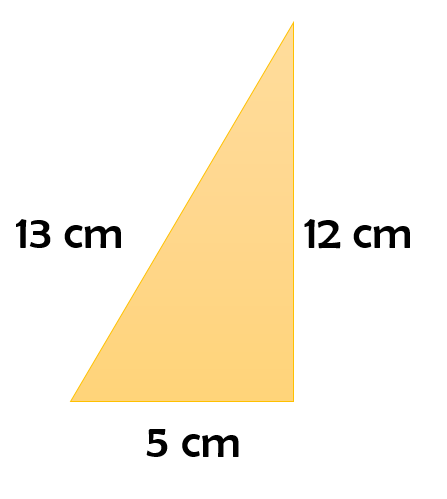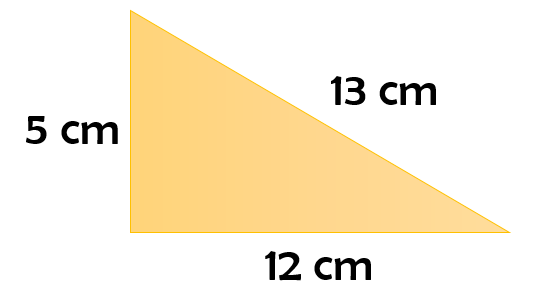# vs.eyeandcontacts.com

## Chapter 13 Surface Areas and Volumes Exercise 13.7

Question 1: Find the volume of the right circular cone with
i) radius 6 cm, height 7 cm
ii) radius 3.5 cm, height 12 cm

i) Given
Radius of the cone = 6 cm
Height of the cone = 7 cm
Volume of the cone = ?
Volume of the cone = 1/3πr²h
= 1/3 × 22/7 × 6 × 6 × 7
= 22 × 2 × 6
= 264 cm³

ii) Given
Radius of the cone = 3.5 cm
Height of the cone = 12 cm
Volume of the cone = ?
Volume of the cone = 1/3πr²h
= 1/3 × 22/7 × 3.5 × 3.5 × 12
= 22 × 0.5 × 3.5 × 4
= 154 cm³

Question 2: Find the capacity in litres of a conical vessel with
i) radius 7 cm, slant height 25 cm
ii) height 12 cm, slant height 13 cm

i) Given
Radius of the cone = 7 cm
Slant height of the cone = 25 cm
Height of the cone = √25² - 7² = √625 - 49 = √576 = 24 cm
Volume of cone = 1/3πr²h
= 1/3 × 22/7 × 7 × 7 × 24
= 22 × 7 × 8
= 1232 cm³
1 l = 1000 cm³
So, 1232 cm³ = 1232/1000 = 1.232 l

ii) Given
Height of the cone = 12 cm
Slant height of the cone = 13 cm
Radius of the cone = √13² - 12² = √169 - 144 = √25 = 5 cm
Volume of cone = 1/3πr²h
= 1/3 × 22/7 × 5 × 5 × 12
= 22/7 × 5 × 5 × 4
= 2200/7 cm³
= 314.28 cm³
1 l = 1000 cm³
So, 314.28 cm³ = 314.28/1000 = 0.31428 l

Question 3: The height of a cone is 15 cm. If its volume is 1570 cm³, find the radius of the base. (Use π = 3.14)

Given
Height of the cone = 15 cm
Volume of the cone = 1570 cm³
Radius of the base of the cone =?
Volume of cone = 1/3πr²h
1570 = 1/3 × 3.14 × r² × 15
1570 = 15.7r²
r² = 1570/15.7
r² = 100
r = √100
r = 10 cm
Therefore, the radius of the base of the cone is 10 cm.

Question 4: If the volume of a right circular cone of height 9 cm is 48π cm³, find the diameter of its base.

Given
Volume of cone = 48π cm³
Height of the cone = 9 cm
Diameter of the base = ?
Volume of the cone = 1/3πr²h
48π = 1/3× π × r² × 9
48π = 3πr²
r² = 48π/3π
r² = 16
r = √16
r = 4 cm
Diameter = 2 × Radius = 2 × 4 = 8 cm
Therefore, the diameter of the base of the cone is 8 cm.

Question 5: A conical pit of top diameter 3.5 m is 12 m deep. What is its capacity in kilolitres?

Given
Diameter of the conical pit = 3.5 m
Height of the conical pit = 12 m
Capacity in kilolitres = ?
Radius = Diameter/2 = 3.5/2 m
Volume of cone = 1/3πr²h
= 1/3 × 22/7 × 3.5/2 × 3.5/2 × 12
= 11 × 0.5 × 3.5 × 2
= 38.5 m³
1 kilolitre = 1 m³
38.5 m³ = 38.5 kl
Therefore, the capacity of the conical pit in kilolitres is 38.5 kl.

Question 6: The volume of a right circular cone is 9856 cm³. If the diameter of the base is 28 cm, find
i) height of the cone
ii) slant height of the cone
iii) curved surface area of the cone

Given
Volume of the cone = 9856 cm³
Diameter of the base of the cone = 28 cm
Height of the cone = ?
Slant height of the cone = ?
Curved Surface Area of the cone = ?
Radius = Diameter/2 = 28/2 = 14 cm
Volume of the cone = 1/3πr²h
9856 = 1/3 × 22/7 × 14 × 14 × h
9856 = 1/3 × 22 × 2 × 14 × h
9856 = 616h/3
h = 9856 × 3/616
h = 48 cm
Slant height of cone = √r² + h²
= √(14)² + (48)²
= √196 + 2304
= √2500
= 50 cm
Curved Surface Area of cone = πrl
= 22/7 × 14 × 50
= 2200 cm²
Therefore, the height of the cone is 48 cm, slant height of the cone is 50 cm and the CSA of the cone is 2200 cm².

Question 7: A right triangle ABC with sides 5 cm, 12 cm and 13 cm is revolved about the side 12 cm. Find the volume of the solid so obtained.If a right angled triangle is revolved, then a cone is obtained.
Given
Radius of the cone = 5 cm
Height of the cone = 12 cm
Volume of the cone = 1/3πr²h
= 1/3 × π × 5 × 5 × 12
= 5 × 5 × 4 × π
= 100π cm³

Question 8: If the triangle ABC in the Question 7 above is revolved about the side 5 cm, then find the volume of the solid so obtained. Find also the ratio of the volumes of the two solids obtained in Questions 7 and 8.Given
Radius of the cone = 12 cm
Height of the cone = 5 cm
Volume of the cone = 1/3πr²h
= 1/3 × π × 12 × 12 × 5
= 5 × 12 × 4 × π
= 240π cm³
Ratio of Volume of cone (Question 7)/Volume of cone (Question 8)
= 100π/240π
= 5/12
= 5:12

Question 9: A heap of wheat is in the form of a cone whose diameter is 10.5 m and height is 3 m. Find its volume. The heap is to be covered by canvas to protect it from rain. Find the area of the canvas required.

Given
Height of the heap = 3 m
Diameter of the heap = 10.5 m
Radius = Diameter/2 = 10.5/2 m
l = √r² + h²
l = √(5.25)²+ (3)²
l = √27.5625 + 9
l = √36.5625
l = 6.04 m (approx)

Volume of the cone = 1/3πr²h
= 1/3 × 22/7 × 10.5/2 × 10.5/2 × 3
= 11 × 1.5 × 10.5/2
= 173.25
= 86.625 m³

Area of the canvas required to cover the heap of wheat = πrl
= 22/7 × 10.5/2 × 6.04
= 11 × 1.5 × 6.04
= 99.66 m²
Therefore, the volume of the heap is 86.625 m³ and the area of the canvas required is 99.66 m².